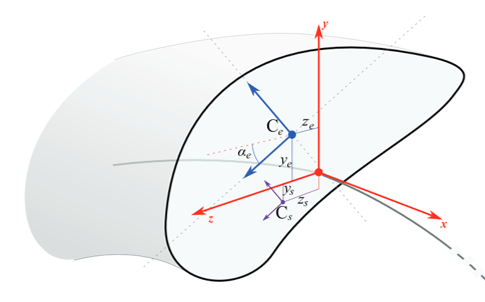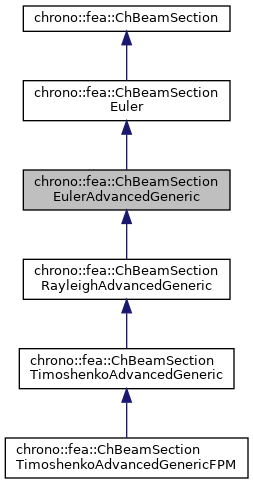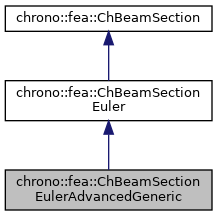## Description

General purpose section of an Euler-Bernoulli beam in 3D, not assuming homogeneous density or homogeneous elasticity, given basic material properties.

This is the case where one uses a FEA preprocessor to compute the rigidity of a complex beam made with multi-layered reinforcements with different elasticity and different density - in such a case you could not use ChBeamSectionEulerAdvanced because you do not have a single E or single density, but you rather have collective values of bending rigidities, and collective mass per unit length. This class allows using these values directly, bypassing any knowledge of area, density, Izz Iyy, E young modulus, etc. To be used with ChElementBeamEuler. The center of mass of the section can have an offset respect to the centerline. This material can be shared between multiple beams.#include <ChBeamSectionEuler.h>[legend][legend]

## Public Member Functions

ChBeamSectionEulerAdvancedGeneric (const double mAx, const double mTxx, const double mByy, const double mBzz, const double malpha, const double mCy, const double mCz, const double mSy, const double mSz, const double mmu, const double mJxx, const double mMy=0, const double mMz=0)

virtual void SetAxialRigidity (const double mv)
Sets the axial rigidity, usually A*E for uniform elasticity, but for nonuniform elasticity here you can put a value ad-hoc from a preprocessor.

virtual void SetXtorsionRigidity (const double mv)
Sets the torsion rigidity, for torsion about X axis, at elastic center, usually J*G for uniform elasticity, but for nonuniform elasticity here you can put a value ad-hoc from a preprocessor.

virtual void SetYbendingRigidity (const double mv)
Sets the bending rigidity, for bending about Y axis, at elastic center, usually Iyy*E for uniform elasticity, but for nonuniform elasticity here you can put a value ad-hoc from a preprocessor.

virtual void SetZbendingRigidity (const double mv)
Sets the bending rigidity, for bending about Z axis, at elastic center, usually Izz*E for uniform elasticity, but for nonuniform elasticity here you can put a value ad-hoc from a preprocessor.

virtual void SetSectionRotation (const double mv)
Set the rotation in [rad], abour elastic center, of the Y Z axes for which the YbendingRigidity and ZbendingRigidity values are defined.

virtual void SetCentroidY (const double mv)
Sets the Y position of the elastic center respect to centerline.

virtual void SetCentroidZ (const double mv)
Sets the Z position of the elastic center respect to centerline.

virtual void SetShearCenterY (const double mv)
Sets the Y position of the shear center respect to centerline.

virtual void SetShearCenterZ (const double mv)
Sets the Z position of the shear center respect to centerline.

virtual void SetMassPerUnitLength (const double mv)
Set mass per unit length, ex.SI units [kg/m] For uniform density it would be A*density, but for nonuniform density here you can put a value ad-hoc from a preprocessor.

virtual void SetInertiaJxxPerUnitLength (const double mv)
Set the Jxx component of the inertia per unit length (polar inertia), computed at centerline. More...

virtual void SetInertiaJxxPerUnitLengthInMassReference (const double mv)
Set inertia moment per unit length Jxx_massref, as assumed computed in the "mass reference" frame, ie. More...

virtual double GetInertiaJxxPerUnitLengthInMassReference ()
Get inertia moment per unit length Jxx_massref, as assumed computed in the "mass reference" frame, ie. More...

void SetCenterOfMass (double my, double mz)
"mass reference": set the displacement of the center of mass respect to the section centerline reference.

double GetCenterOfMassY ()

double GetCenterOfMassZ ()

virtual double GetAxialRigidity () const override
Gets the axial rigidity, usually A*E, but might be ad hoc.

virtual double GetXtorsionRigidity () const override
Gets the torsion rigidity, for torsion about X axis at elastic center, usually J*G, but might be ad hoc.

virtual double GetYbendingRigidity () const override
Gets the bending rigidity, for bending about Y axis at elastic center, usually Iyy*E, but might be ad hoc.

virtual double GetZbendingRigidity () const override
Gets the bending rigidity, for bending about Z axis at elastic center, usually Izz*E, but might be ad hoc.

virtual double GetSectionRotation () const override
Set the rotation of the Y Z section axes for which the YbendingRigidity and ZbendingRigidity are defined.

virtual double GetCentroidY () const override
Gets the Y position of the elastic center respect to centerline.

virtual double GetCentroidZ () const override
Gets the Z position of the elastic center respect to centerline.

virtual double GetShearCenterY () const override
Gets the Y position of the shear center respect to centerline.

virtual double GetShearCenterZ () const override
Gets the Z position of the shear center respect to centerline.

virtual void ComputeInertiaMatrix (ChMatrixNM< double, 6, 6 > &M) override
Compute the 6x6 sectional inertia matrix, as in {x_momentum,w_momentum}=[Mm]{xvel,wvel}.

virtual void ComputeInertiaDampingMatrix (ChMatrixNM< double, 6, 6 > &Ri, const ChVector<> &mW) override
Compute the 6x6 sectional inertia damping matrix [Ri] (gyroscopic matrix damping) More...

virtual void ComputeInertiaStiffnessMatrix (ChMatrixNM< double, 6, 6 > &Ki, const ChVector<> &mWvel, const ChVector<> &mWacc, const ChVector<> &mXacc) override
Compute the 6x6 sectional inertia stiffness matrix [Ki^]. More...

virtual void ComputeQuadraticTerms (ChVector<> &mF, ChVector<> &mT, const ChVector<> &mW) override
Compute the centrifugal term and gyroscopic term. More...

virtual double GetMassPerUnitLength () const override
Get mass per unit length, ex.SI units [kg/m].

virtual double GetInertiaJxxPerUnitLength () const override
Get the Jxx component of the inertia per unit length (polar inertia), at centerline.Public Member Functions inherited from chrono::fea::ChBeamSectionEuler
virtual void ComputeInertialForce (ChVector<> &mFi, ChVector<> &mTi, const ChVector<> &mWvel, const ChVector<> &mWacc, const ChVector<> &mXacc)
Compute the total inertial forces (per unit length). More...

void SetArtificialJyyJzzFactor (double mf)
The Euler beam model has no rotational inertia per each section, assuming mass is concentrated on the centerline. More...

double GetArtificialJyyJzzFactor ()

virtual void SetBeamRaleyghDampingAlpha (double malpha)
Set the "alpha" Rayleigh damping ratio,
the mass-proportional structural damping in: R = alpha*M + beta*K

double GetBeamRaleyghDampingAlpha ()

virtual void SetBeamRaleyghDampingBeta (double mbeta)
Set the "beta" Rayleigh damping ratio, the stiffness-proportional structural damping in: R = alpha*M + beta*K

double GetBeamRaleyghDampingBeta ()

virtual void SetBeamRaleyghDamping (double mbeta, double malpha=0)
Set both beta and alpha coefficients in Rayleigh damping model: R = alpha*M + beta*K. More...Public Member Functions inherited from chrono::fea::ChBeamSection
void SetDrawShape (std::shared_ptr< ChBeamSectionShape > mshape)
Set the graphical representation for this section. More...

std::shared_ptr< ChBeamSectionShapeGetDrawShape () const
Get the drawing shape of this section (i.e.a 2D profile used for drawing 3D tesselation and visualization) By default a thin square section, use SetDrawShape() to change it.

void SetDrawThickness (double thickness_y, double thickness_z)
Shortcut: adds a ChBeamSectionShapeRectangular for visualization as a centered rectangular beam, and sets its width/height. More...

Shortcut: adds a ChBeamSectionShapeCircular for visualization as a centered circular beam, and sets its radius. More...

void SetCircular (bool ic)
OBSOLETE only for backward compability

## Protected Attributes

double Ax

double Txx

double Byy

double Bzz

double alpha

double Cy

double Cz

double Sy

double Sz

double mu

double Jxx

double My

double MzProtected Attributes inherited from chrono::fea::ChBeamSectionEuler
double rdamping_beta

double rdamping_alpha

double JzzJyy_factorPublic Attributes inherited from chrono::fea::ChBeamSectionEuler
bool compute_inertia_damping_matrix = true
Flag that turns on/off the computation of the [Ri] 'gyroscopic' inertial damping matrix. More...

bool compute_inertia_stiffness_matrix = true
Flag that turns on/off the computation of the [Ki] inertial stiffness matrix. More...

bool compute_Ri_Ki_by_num_diff = false
Flag for computing the Ri and Ki matrices via numerical differentiation even if an analytical expression is provided. More...

## Constructor & Destructor Documentation

 chrono::fea::ChBeamSectionEulerAdvancedGeneric::ChBeamSectionEulerAdvancedGeneric ( const double mAx, const double mTxx, const double mByy, const double mBzz, const double malpha, const double mCy, const double mCz, const double mSy, const double mSz, const double mmu, const double mJxx, const double mMy = 0, const double mMz = 0 )
inline
Parameters
 mAx axial rigidity mTxx torsion rigidity mByy bending regidity about yy mBzz bending rigidity about zz malpha section rotation about elastic center [rad] mCy elastic center y displacement respect to centerline mCz elastic center z displacement respect to centerline mSy shear center y displacement respect to centerline mSz shear center z displacement respect to centerline mmu mass per unit length mJxx polar inertia Jxx per unit lenght, measured respect to centerline mMy mass center y displacement respect to centerline mMz mass center z displacement respect to centerline

## Member Function Documentation

 void chrono::fea::ChBeamSectionEulerAdvancedGeneric::ComputeInertiaDampingMatrix ( ChMatrixNM< double, 6, 6 > & Ri, const ChVector<> & mW )
overridevirtual

Compute the 6x6 sectional inertia damping matrix [Ri] (gyroscopic matrix damping)

Parameters
 Ri 6x6 sectional inertial-damping (gyroscopic damping) matrix values here mW current angular velocity of section, in material frame

Reimplemented from chrono::fea::ChBeamSectionEuler.

## ◆ ComputeInertiaStiffnessMatrix()

 void chrono::fea::ChBeamSectionEulerAdvancedGeneric::ComputeInertiaStiffnessMatrix ( ChMatrixNM< double, 6, 6 > & Ki, const ChVector<> & mWvel, const ChVector<> & mWacc, const ChVector<> & mXacc )
overridevirtual

Compute the 6x6 sectional inertia stiffness matrix [Ki^].

Parameters
 Ki 6x6 sectional inertial-stiffness matrix [Ki^] values here mWvel current angular velocity of section, in material frame mWacc current angular acceleration of section, in material frame mXacc current acceleration of section, in material frame (not absolute!)

Reimplemented from chrono::fea::ChBeamSectionEuler.

 void chrono::fea::ChBeamSectionEulerAdvancedGeneric::ComputeQuadraticTerms ( ChVector<> & mF, ChVector<> & mT, const ChVector<> & mW )
overridevirtual

Compute the centrifugal term and gyroscopic term.

Parameters
 mF centrifugal term (if any) returned here mT gyroscopic term returned here mW current angular velocity of section, in material frame

Implements chrono::fea::ChBeamSectionEuler.

## ◆ GetInertiaJxxPerUnitLengthInMassReference()

inlinevirtual

Get inertia moment per unit length Jxx_massref, as assumed computed in the "mass reference" frame, ie.

centered at the center of mass

## ◆ SetInertiaJxxPerUnitLength()

 virtual void chrono::fea::ChBeamSectionEulerAdvancedGeneric::SetInertiaJxxPerUnitLength ( const double mv )
inlinevirtual

Set the Jxx component of the inertia per unit length (polar inertia), computed at centerline.

For uniform density it would be Ixx*density or, by polar theorem, (Izz+Iyy)*density, but for nonuniform density here you can put a value ad-hoc from a preprocessor

## ◆ SetInertiaJxxPerUnitLengthInMassReference()

 virtual void chrono::fea::ChBeamSectionEulerAdvancedGeneric::SetInertiaJxxPerUnitLengthInMassReference ( const double mv )
inlinevirtual

Set inertia moment per unit length Jxx_massref, as assumed computed in the "mass reference" frame, ie.

centered at the center of mass. Call this after you set SetCenterOfMass() and SetMassPerUnitLength()

The documentation for this class was generated from the following files:
• /builds/uwsbel/chrono/src/chrono/fea/ChBeamSectionEuler.h
• /builds/uwsbel/chrono/src/chrono/fea/ChBeamSectionEuler.cpp